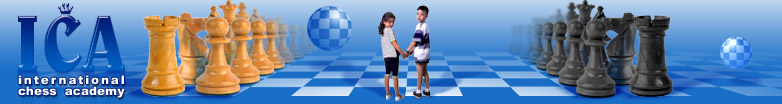HomeChess CampsPrivate LessonsICA TournamentsScheduleICA staffBest studentsAchievementsChessKid (ICA Club)Chess newsMath OlympiadPhoto galleryLinksChess In The SchoolContact us
 Why Teach Spatial Thinking Skills And How It Relates To The Singapore Posted: 8/12/2015

# Why teach spatial thinking skills and how it relates to the Singapore math system.

We asked children as old as 4th grade (who were not prepped in the Singapore math system) to solve a simple problem. "Nina has 10 pencils and Kira has 4 pencils. How many pencils should Nina give Kira so that they have an equal number of pencils?"
To our surprise only a few children were able to get a correct answer and none of those used spatial thinking skills such as drawing a model to represent the numbers given in the problem. The strategy the children used was simple "trial and error". Of course, after getting help from an adult (teacher or parent) the children understood the other strategy too.  This solves the problem in 3 steps. First add, 10 + 4= 14 (total number of pencils that both girls have). Then, divide 14 by 2 to get 7, the number of pencils each girl would have after the exchange. Third, subtract 7 from 10 to find the number of pencils that Nina must give Kira, 3 pencils, in order for both girls to have an equal number of pencils.
Parents and even educators do not know about the Singapore math system. They learned math without it and they teach their children to solve problems such as the one above using either one of the strategies above.
We advocate the Singapore math system because it employs spatial thinking in every strategy that it uses to teach math. For example, to solve the problem above this math system teaches to draw a model to represent the objects in the problem. Of course, for this method a child needs to develop an understanding that any abstract number can be represented in a model of units. For instance, in this particular problem the verbal description must be interpreted in such a way where Nina would be represented with a longer bar labeled 10, and Kira would be represented with a shorter bar labeled 4. Then, a child needs to visualize the difference in the lengths of the 2 bars in such a way that it is also a difference in the number of pencils, 6. Lastly, in order to figure out how many pencils Nina must give Kira to keep it equal, a difference in length must be divided in half, thus the number of pencils representing the difference, 6, must be split in half to obtain the same answer as we have already described above (Nina must give Kira 3 pencils). Although, drawing a model takes time to learn, it may still be more appealing to children because it is visual and takes only 2 steps.
In summary, we must help children develop an ability to construct models to create mental images of objects from verbal descriptions in the word problem.  In addition, we should use the Singapore math system to help children develop those skills because this visual representation strategy will appeal to children for at least three reasons:
* Children will develop an ability to do challenging math without any help from adults
* Children will learn a shorter way of solution and it would make them feel good!
* It will prepare children for geometry and algebra in the higher grades